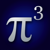# Preprogrammed equations

Pi Cubed ships with over 150 preprogrammed equations. These equations are drawn from a wide range of scientific and engineering disciplines, as well as from everyday applications. The library provides both a quick reference for these equations and a means of quickly performing their calculations.

To access the library from the main math entry screen on the iPhone, tap on the book icon in the upper right of the screen. On the iPad, the library will be displayed either to the left of the screen (in landscape orientation) or in a popover (in portrait).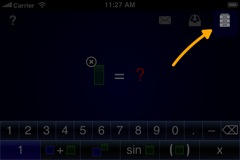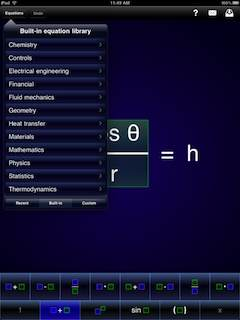You will be presented with the main library interface. Initially, the list of recent calculations will be selected. You can proceed to the built-in equation library by tapping on the tab labeled "Built-in library" on the iPhone, or the "Built-in" toolbar option on the iPad.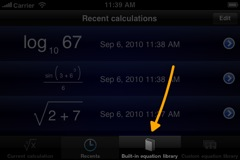This will bring you to the main list of equation categories.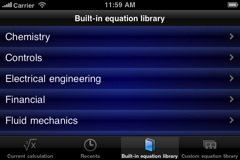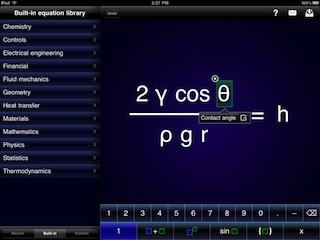Tapping on an item will take you to its respective category of equations.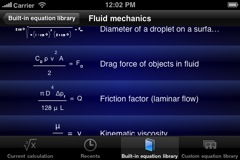Within a category, you will see a list of equations with each entry comprised of a thumbnail image of the equation along with its description. Tapping on an equation will select it to be loaded from the database.

Once you have loaded an equation, you can work with it as you would for any standard mathematical calculation. Tapping on a variable will bring up a label that describes the purpose of that variable. This can serve as a useful reference or memory aid.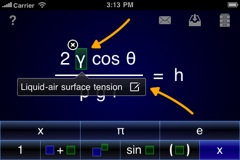Additionally, tapping on the result of the equation will bring up a description for that as well.As you start typing a numerical value for a variable, any other instances of that variable in the equation will be changed to match. When all variables have values assigned to them, the result variable will display the calculated answer to the equation.

Like with numerical calculations, you can send a plain text, LaTeX, or PDF representation of the equation via the Mail application by tapping on the appropriate icon at the top of the screen.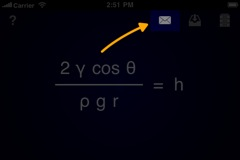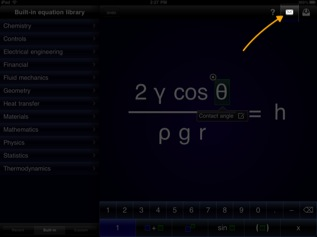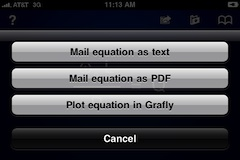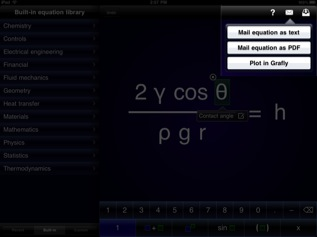### Equation categories

The categories of equations within Pi Cubed include:

#### Chemistry

These equations cover chemical reactions, reaction kinetics, solubility, pH, and gas behavior.

#### Controls

These equations deal with control systems, primarily proportional-integral-derivative (PID) controllers.

#### Electrical engineering

These equations deal with the properties of electrical circuits, from resistance and capacitance to resonances.

#### Financial

These equations revolve around interest calculations and the time value of money.

#### Fluid mechanics

These equations cover fluid behavior, including surface tension and capillarity, laminar and turbulent flow, drag force, pressure, and the speed of sound.

#### Geometry

These equations include the volumes and surface areas of common shapes, as well as the Pythagorean theorem.

#### Heat transfer

These equations govern heat transfer by conduction through objects, by convection off of a surface, or by radiation from a body.

#### Materials

These equations cover stress and strain, thermal deformation, and fundamental materials science.

#### Mathematics

These are equations handling more generic mathematical cases, such as polar coordinates and polynomial roots.

#### Physics

These equations govern areas in fundamental physics such as straight-line motion, kinetic energy, projectile motion, and vibration.

#### Statistics

These equations include a few simple statistical concepts.

#### Thermodynamics

These equations cover heat engine efficiencies, as well as work and entropy in some simple systems.